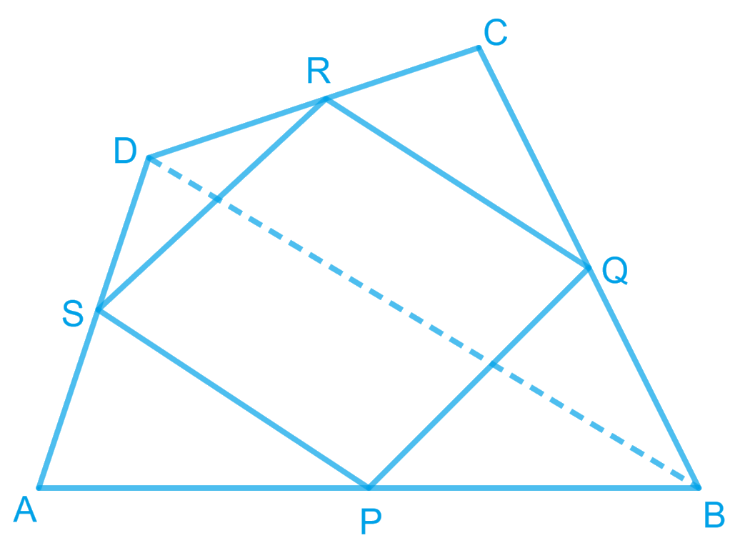In the verge of coronavirus pandemic, we are providing FREE access to our entire Online Curriculum to ensure Learning Doesn't STOP!

# Ex.8.2 Q6 Quadrilaterals Solution - NCERT Maths Class 9

Go back to  'Ex.8.2'

## Question

Show that the line segments joining the mid-points of the opposite sides of a quadrilateral bisect each other.

Video Solution
Ex 8.2 | Question 6

## Text Solution

What is known?

$$ABCD$$ is a quadrilateral in which $$P, Q, R,$$ and $$S$$ are the mid-points of sides $$AB, BC, CD,$$ and $$DA$$ respectively.

What is unknown?

How we can show that the line segments joining the mid-points of the opposite sides of a quadrilateral bisect each other.

Reasoning:

In a triangle, the line segment joining the mid-points of any two sides of the triangle is parallel to the third side and is half of it. In a quadrilateral if one pair of opposite sides is parallel and equal to each other then it is a parallelogram.

Steps:Let $$ABCD$$ is a quadrilateral in which $$P$$, $$Q$$, $$R$$, and $$S$$ are the mid-points of sides $$AB$$, $$BC$$, $$CD$$, and $$DA$$ respectively. Join $$PQ$$, $$QR$$, $$RS$$, $$SP$$, and $$BD$$.

In $$\Delta {ABD,}$$ $$S$$ and $$P$$ are the mid-points of $$AD$$ and $$AB$$ respectively. Therefore, by using mid-point theorem, it can be said that

\begin{align} SP \parallel BD \; and\; SP\!=\!\frac{1}{2} BD \quad\!\!... (1) \end{align}

Similarly, in $$\Delta$$ $$BCD$$,

\begin{align} QR \parallel BD \; and\; QR \!=\!\frac{1}{2} BD \quad\!\!... (2) \end{align}

From Equations (1) and (2), we obtain

$$SP$$ $$\parallel$$$$QR$$ and $$SP = QR$$

In quadrilateral $$SPQR$$, one pair of opposite sides is equal and parallel to each other. Therefore, $$SPQR$$ is a parallelogram.

We know that diagonals of a parallelogram bisect each other.

Hence, $$PR$$ and $$QS$$ bisect each other.

Video Solution